Student names:_________________________________________

CM 440/667

Practical: Voltammetry Simulation

Students who are scheduled to do the polarography prac prior to this date should attempt the simulation before doing the prac - it will greatly enhance your understanding of the prac.

The program Polar41c has been downloaded onto the computers in the  Department's computer lab.  You can download a copy yourself (as a zip file) from http://www.DrHuang.com and install it on your own computer.

In this exercise you will be given some information about voltammetry and asked to predict the outcome of an experiment.  You will then perform the experiment on the simulator, compare the outcome with your prediction, and think about any discrepancies.  You will not receive extra marks for making the 'right' prediction.  Rather, I will be looking for evidence of your engagement with the process of prediction, comparison, and reflection.  You may work in pairs or a small group, discussing your predictions and any reasons for discrepancies between them and the outcome of the simulation.  Don’t feel too constrained by these notes – feel free to explore the simulation along the way, doing additional experiments if you wish (a couple of proformas are attached to the end of this document to record the results of any such experiments.)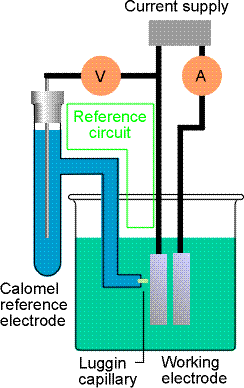DC Voltammetry

In voltammetry, an inert electrode (eg., Pt, C, Hg) is placed in a solution containing electroactive species.  (Polarography is a subset of voltammetry that uses Hg electrodes.)  The potential of the electrode is changed as a function of time.

If an electrochemical reaction occurs, electrons are transferred and a current flows.   The current is measured as a function of the potential applied to the electrode.  A voltammogram is a plot of the current, i, on the vertical axis versus the potential, E, on the horizontal axis.  Cathodic (reduction) currents are shown in the positive y-direction and negative potentials are shown in the positive x-direction

In this series of experiments we are looking at the two-electron reduction of Cu2+ to Cu.  The Eo of the reduction is +0.34 V relative to the standard hydrogen electrode, or +0.10 V relative to the saturated calomel electrode (which is more commonly used as a reference in voltammetry).

If we apply a potential of greater (more positive) than 0.10 V, the reaction

Cu2+ + 2e  ®  Cu

will not occur.  In voltammetry of metal ions, we start with an applied potential well in excess (more positive) of the Eo of the metal ion present and scan the potential in the negative direction as a linear function of time.  What do you think the voltammogram (i versus E) will look like as the potential approaches and passes the Eo?  Remember there are no marks for getting it right - just give it a go!

·        Start/ Programs/ Polar/ Polar

·        Maximise

Now run the simulation:

·        Input/ Techniques ® DC voltammetry

·        Input/ Mechanism ® A + 2e®B

·        Run/ Simulate

How does the simulated voltammogram compare with your prediction?  Did you predict the gradual increase of current with voltage around the Eo?  Current (coulombs per second) is a measure of the rate of the electrochemical reaction.  The reaction starts slowly at first but is driven faster as the voltage is increased.  Did you predict the levelling off of the current or did you have the current increasing infinitely?  Can you offer an explanation for the current plateau?

As Cu2+ ions near the surface of the electrode react to form Cu (that is deposited on to the electrode), the region close to the electrode is depleted of Cu2+ ions.  We have

to wait for more Cu2+ ions to diffuse from the bulk solution (where they are in high concentration) to the electrode surface (where they are in low concentration) before the reaction can continue. The rate of reaction, and hence the current, becomes limited by the rate of diffusion of ions from the bulk of the solution to the electrode surface, and the current plateaus.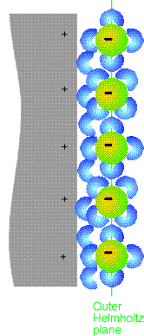There are a couple of parameters that can be obtained from the voltammogram that are of interest to us.  What do you think these might be?  Draw them on a voltammogram:

Find out what they are:

·        Analysis/ Find Halfwave E

Can you see where these are on your simulated voltammogram?  You can put gridlines in if you want:

·        Display/ Option ® Grid

The diffusion current , id, is the (diffusion) limited current at the top of the voltammogram.  The half-wave potential, E1/2, is the potential at id/2.

What effect do you predict changing the concentration of the Cu2+ ions will have on the voltammogram?  Sketch a voltammogram for the 1 x 10-3 M solution you have already studied, and for a 2 x 10-3 M solution.

Now run the simulation:

·        Display/ Option ® Overlap (this enables the two voltammograms to be displayed on the same plot)

·        Input/ Chemicals ® Canal(A) = 2e-3

·        Run/ Simulate

What has happened to id and E1/2?  Find out:

·        Analysis/ Find Halfwave E

You may well have expected to see a change in E1/2 with concentration since this is what is seen in potentiometry (as described by the Nernst equation).  In voltammetry, E1/2 is unchanged by concentration, and it is id that is concentration dependent.  Try another couple of concentrations, then plot id versus concentration in the space below.  What do you notice?

The voltammogram for a copper solution of unknown concentration run under the same conditions has id = 7 x 10-4 A.  What is the concentration of the solution?

The diffusion-limited current is concentration dependent and so can be used, as above, as an analytical probe to determine the concentration of an unknown copper solution.  To ensure the concentration dependence of the limiting current, we have to ensure that the Cu2+ ions move by diffusion (under the influence of a concentration gradient) and not by convection (stirring) or migration (under the influence of an electric field).  Since we are applying a potential across the electrodes, the ions will move by migration – a positively charged ion will be attracted to a negatively charged electrode.  To minimise the migration of the electroactive species, we add a large excess of electroinactive supporting electrode to the solution.  The electroinactive ions move by migration, but the electroactive Cu2+  ions move predominantly by diffusion so the Faradaic current is proportional to concentration.

The linear relationship between id and concentration can be used as an analytical tool to determine the concentration of an electroactive species in solution.  But how do we know what electroactive species is/are present?  It's the value of E1/2 that can indicate this.  As you know, redox couples have distinct reduction potentials.  The half-wave potential, E1/2 is very closely related to the Eo values.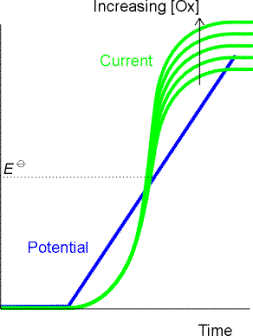What do you think that the voltammogram for the reduction of Pb2+ (Eo = - 0.40 V vs. SCE) will look like?  Draw voltammograms for Cu2+ and Pb2+ on the same axes.

·        Display/ Option ® Overlap off

·        Input/ Chemicals ® Canal(A) = 1e-3

·        Input/ Instrument  ® Eend = -0.6 V

·        Run/ Simulate

·        Display/ Option ® Overlap on

·        Input/ Chemicals Eo = - 0.40

·        Run/ Simulate

·        Analysis/ Find Halfwave E

Was your prediction right?  E1/2 can be used for identification.

Next we look at the effect of changing the number of electrons (n) involved in the reduction process.  What do you predict will happen to the voltammogram as you increase the number of electrons?  Will the current that flows increase, decrease, or stay the same?  Will the diffusion limited current, id, increase, decrease, or stay the same?  Will there be any effect on E1/2?  Draw a series of voltammograms for n = 1, 2 and 3.

Now do a series of experiments and see how your prediction measures up:

·        Display/ Option ® Overlap off

·        Input/ Techniques ® DC voltammetry

·        Input/ Chemicals ® Eo = 0.1

·        Input/ Instrument  ® Eend = - 0.3 V

·        Input/ Mechanism ® A + 1e®B (default setting)

·        Run/ Simulate

·        Display/ Option ® Overlap on

·        Input/ Mechanism ® A + 2e®B

·        Run/ Simulate

·        Input/ Mechanism ® A + 3e®B

·        Run/ Simulate

·        Analysis/ Find Halfwave E

Describe the effect on the shape of the voltammogram, the diffusion-limited current, id, and the half-wave potential, E1/2? Is that what you were expecting?

The diffusion limited current, id, increases linearly with n.  The diagram opposite is normalised (i/id) and does not show this.

The shape of the voltammogram is given by the equation:

E = E1/2 + (0.0592/n)*log [(id - i)/i]

So, as n is increased the voltammetric wave (i versus E) becomes steeper.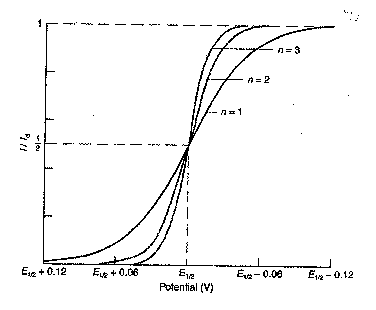What effect might varying the diffusion coefficient, D, of the metal ion have?  (The diffusion coefficient could change as a  result of a change in temperature or a change of solvent.)  An increase in the diffusion coefficient means that the metal ion can move from the bulk solution to the surface of the electrode faster.  What effect would this have on the limiting current, id?  What effect would there be on E1/2?  Draw a series of voltammograms for which D = 1x 10-5, 4 x 10-5, and 9 x10-5  cm2/s.

Now do the experiment:

·        Display/ Option ® Overlap off

·        Input/ Mechanism ® A + 2e®B

·        Input/ Chemicals ® Canal = 1e-3

·        Input/ Chemicals  D = 1e-5 (default setting)

·        Run/ Simulate

·        Display/ Option ® Overlap on

·        Input/ Chemicals ® D = 4e-5 for A.  Keep D for B at 1e-5.

·        Run/ Simulate

·        Input/ Chemicals ® D = 9e-5 for A.  Keep D for B at 1e-5.

·        Run/ Simulate

·        Analysis/ Find Halfwave E

Can you see a relationship between id and D?  What is it?  Is it what you expected?

You were probably expecting id to increase as you increased D, but you would probably not have expected the D1/2 relationship.  More about that in lectures.

Were you surprised by the dependence of E1/2 on D?  Try plotting E1/2 versus log D on the grid overleaf.  What do you conclude?

E1/2 depends on log D.  Again, more in lectures.

Are  there any other experiments that you would like to do?  A couple of proformas are attached to the end of this document to record your aims, settings, predictions, results and discussions.

Normal Pulse Voltammetry

Normal pulse (NP) voltammetry replaces the DC voltage ramp (the linear change of potential with time) with voltage pulses of increasing amplitude.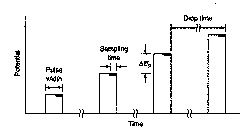The current is sampled near the end of the pulse when the Faradaic current (due to the reduction of Cu2+) is large compared to the charging current (due to the migration of the electroinactive supporting electrolyte ions in response to the charging of the electrode) – ie., the signal-to-noise ratio is largest.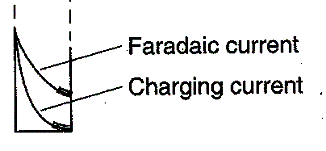What do you predict that the resulting voltammogram will look like?  Draw a DC voltammogram and the corresponding NP voltammogram.

·        Display/ Option ® Overlap off

·        Input/ Chemicals ® D = 1e-5

·        Run/ Simulate

·        Display/ Option ® Overlap on

·        Input/ Techniques ® Normal pulse voltammetry

·        Run/ Simulate

·        Analysis/ Find Halfwave E

Were you expecting a similar shape wave?  Has E1/2 moved?  How do the id's compare?  Because the current is sampled near the end of the pulse when the Faradaic current (due to the reduction of Cu2+) is large compared to the charging current (due to the migration of ions in response to the charging of the electrode), the signal is increased (as you can see).  But also the noise is decreased and hence the signal-to-noise ratio is improved by a factor of about 10.  The detection limit of NP voltammetry is 10-6 M, compared with 10-5 M for DC.

Differential Pulse Voltammetry

In differential pulse (DP) voltammetry, small pulses are superimposed on a linear voltage ramp.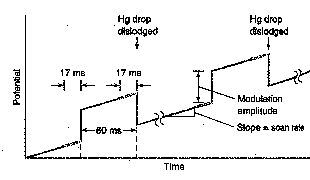Current is measured before and after the application of the pulse and the difference in current is recorded as a function of the applied voltage.  The resulting voltammogram is nearly the derivative of a DC voltammogram.  Can you predict the shape of the voltammogram?  Consider the size of Di as you move in small, constant DE steps along a DC polarogram.  Where is Di a maximum?

·        Display/ Option ® Overlap off

·        Input/ Techniques ® DC voltammetry

·        Run/ Simulate

·        Display/ Option ® Overlap on

·        Input/ Techniques ® Differential pulse voltammetry

·        Run/ Simulate

·        Analysis/ Find Peak

Did you predict the peak shape?  What peak parameter do you think is most likely to be used in place of E1/2 to identify an electroactive species?  What parameter do you expect to be related to the concentration of the electroactive species?  Indicate these parameters on a voltammogram.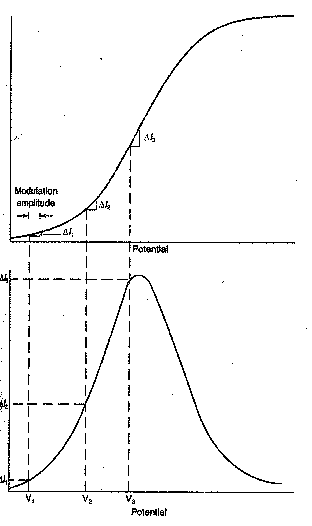Run a series of experiments to test that the peak current, ip, and the peak area are proportional to concentration, c.  Graph ip  and peak area versus c.

The magnitude of the pulse that is superimposed on the DC ramp in DP voltammetry can be changed.  What effect would increasing the size of this voltage pulse have on driving the reaction and hence on the current?  Might any other peak parameters be affected?  Sketch some voltammograms:

Well, try it and see:

·        Display/ Option ® Overlap off

·        Input/ Chemicals ® Canal = 1e-3

·        Input/ Instrument ® Epulse = - 0.05 (default setting)

·        Run/ Simulate

·        Display/ Option ® Overlap on

·        Input/ Instrument ® Epulse = - 0.02

·        Run/ Simulate

·        Input/ Instrument ® Epulse = - 0.01

·        Run/ Simulate

·        Analysis/ Find Peak

You were probably expecting to see an increase in the signal, but what else do you notice?  Estimate the peak width (in volts) at half-height.  To help you do this, you could insert gridlines and/or print the voltammograms and measure the peak widths at half height with a ruler.  What do you notice?  What implications does this have for resolution of neighbouring peaks?

DP voltammetry allows an even greater increase in the signal to noise ratio and the detection limit is of the order of 10-7 M.  Because it is easier to distinguish partially overlapping peaks than partially overlapping waves, resolution is improved (provided too large a pulse is not used) from 0.2 V for DC and NP to 0.05 V for DP voltammetry.

Square Wave Voltammetry

In square wave (SQ) voltammetry, an alternating (positive, negative, positive, negative….) wave that is square in shape is superimposed on a voltage staircase.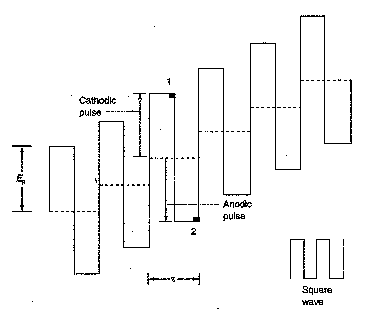The reverse (anodic) pulse causes re-oxidation of the product (Cu(s)) of each forward (cathodic) pulse.  The voltammetric signal is the difference between these two signals and hence will only be recorded when significant amounts of the oxidised (Cu2+) and reduced forms (Cu(s)) are present.  Can you predict the shape of the SQ voltammogram?

Try it and see:

·        Display/ Option ® Overlap off

·        Input/ Techniques ® Square Wave voltammetry

·        Run/ Simulate

Square wave voltammetry is about 5 times more sensitive than differential pulse.  It is also about 100 times faster - an entire square wave voltammogram can be obtained in less than one second.  It can be used for the detection of compounds emerging from a liquid chromatograph.  Compounds are distinguished by their retention times and E1/2 and are quantified by peak height or area.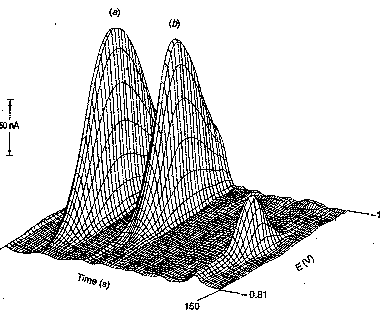Stripping Analysis

In stripping analysis, Cu2+ is concentrated onto the electrode (as Cu) by reduction at a potential more negative (why?) than E1/2.  The copper metal is then stripped off the electrode by reversing the voltage  - ie., sweeping in a positive direction - driving the oxidation reaction.  The current measured during the oxidation is related to the amount of Cu initially deposited which, under a given set of experimental conditions, is related to the concentration of Cu2+.  Predict the shape of a DC stripping voltammogram.  How will the current measured compare with that for a normal DC voltammogram?  Think about both the signs and magnitudes of the currents.

Run the simulation:

·        Display/ Option ® Overlap off

·        Input/ Techniques ® DC voltammetry

·        Run/ Simulate

·        Display/ Option ® Overlap on

·        Input/ Instrument ® Pre-concentration on.  Note that the potential will be held at - 0.3 V for 600 s before being reversed and swept in the positive direction.

·        Run/ Simulate

·        Analysis/ Find Halfwave E

Stripping is the most sensitive of voltammetric techniques because the analyte is concentrated from dilute solution.  The longer the period of concentration, the more sensitive the analysis.  Confirm this:

·        Input/ Instrument ® tpre = 1200 s

·        Run/ Simulate

·        Analysis/ Find Halfwave E

Detection limits are ca. 10-10 M

Linear Sweep Voltammetry

Linear sweep voltammetry (LSV) is similar to DC voltammetry - a linear voltage ramp is applied to the electrode - except that the rate of sweep is much greater.   Do you expect the LS voltammogram to be the same as the DC?  Think about what would happen at the surface of the electrode as the voltage is rapidly increased.  Would new Cu2+ ions have sufficient time to move from the bulk solution to replace those that are reduced?  Sketch the waveform that you expect for LSV.

Now do the experiment

·        Display/ Option ® Overlap off

·        Input/ Techniques ® DC voltammetry

·        Input/ Instrument ® Pre-concentration off

·        Run/ Simulate

·        Display/ Option ® Overlap on

·        Input/ Techniques ® Linear sweep and cyclic voltammetry

·        Run/ Simulate

·

Instead of levelling off at the top of the LSV wave, the current decreases as the voltage is further increased.  This happens because Cu2+ ions become depleted near the surface of the electrode and movement from the bulk solution is too slow to replenish them.

Perform a series of experiments to confirm that the peak current, ip, is proportional to concentration.  Plot an appropriate graph below.

Cyclic Voltammetry

In cyclic voltammetry (CV), the LSV voltage ramp is reversed to bring the potential back to its initial value.  When the potential becomes sufficiently less negative, Cu is oxidised back to Cu2+.  The process of reduction and re-oxidation can be repeated many times.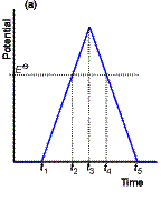Predict the shape of the resulting waveform:

Run the experiment

·        Display/ Option ® Overlap off

·        Input/ Techniques ® Linear sweep and cyclic voltammetry

·        Input/ Instrument ® Set "scan" to cycle

·        Run/ Simulate

Did you predict the anodic and cathodic waves?  Did you predict that ipa = - ipc?  Did you predict the separation of the voltages at which the anodic and cathodic peaks occur?  For a reversible reaction (one that is fast enough to maintain equilibrium concentrations of reactant and product at the electrode surface):

Epa - Epc = 0.057 / n

where n is the number of electrons.  Is the Cu2+ + 2e ® Cu(s) reaction reversible?  Check it out:

·        Analysis/ Find peak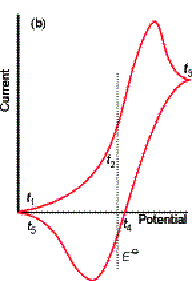For an irreversible reaction, the peaks are more drawn out and separated.  Where the oxidation is very slow, no anodic peak is seen.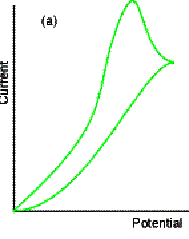Aim:

Settings:

Prediction:

Results:

Discussion:

Aim:

Settings:

Prediction:

Results:

Discussion: